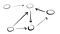# Solving Minimum Coin Change

For many people that are preparing for coding interviews, the fear of dynamic programming (DP) questions is quite common. This is in part due to the type of problems that often requires DP to solve. These questions often concern combinatorics and repetitive calculations.

Now, if someone was learning about DP, it’s likely that they would come across the minimum coin change problem (or at least a variant of it). In this post, we’ll discuss the logic involved in solving this problem.

But before we can dive into the problem, let’s talk a bit about what dynamic programming is.

Here’s a…

# Algorithms on Graphs: Heaps Demystified

Much of the algorithms we’ll be discussing in this series will require us to efficiently pull the lowest values in a set of unsorted numbers without looking at every number in the list.

While sorting or traversing a list may appear to be efficient, it gets rather expensive when we have to do these multiple times, especially when we’re constantly updating or adding new elements to the list.

This is where heaps come into play in helping us get the lowest (or highest) values in a much faster way and in this post, we’ll discuss how.

# What is a heap?

First, let’s talk discuss…

# Algorithms on Graphs: Simplifying DAGs with Topological Sort

One of the difficulties in dealing with directed acyclic graphs (DAGs) is that they can look complicated and messy. This makes it difficult to see the relationships that vertices have with each other.

In this post, we’re going to discuss and learn how to rearrange the vertices of DAGs in such a way that will allow us to simplify these graphs and see possible patterns much easier.

# Source and Sink Vertices

Before we start, we need to be familiar with some terms: source and sink vertices.

## Source Vertex

A source vertex is simply a vertex where no edges point towards it. For example:

Vertex A has…

# Algorithms on Graphs: Directed Graphs and Cycle Detection

By now, we have an understanding of what a graph is and learned some of the methods in traversing them.

While, we’ve already seen directed graphs, it’s time to get a formal understanding of them and uncover exciting properties that come along with their nature.

In this post, we’ll be exploring directed graphs along with learning how to determine if they have cycles within them.

Let’s first start off with learning what a directed graph is.

# Directed Graphs

Directed graphs are graphs where edges go in one direction. Here’s an example.Notice how every edge is pointing in one direction to another vertex

As we can see, we use arrows to indicate that an…

# Algorithms on Graphs: Let’s talk Depth-First Search (DFS) and Breadth-First Search (BFS)

One thing that we’ll be doing a lot with the algorithms in this series is graph traversal. What does this mean exactly?

Basically, the idea is that we’ll be moving around the graph from one vertex to another and discovering the properties of their interconnected relationships.

Two of the most commonly used algorithms that we’ll use a lot is: Depth-First Search (DFS) and Breadth-First Search (BFS).

While both these algorithms allow us to traverse graphs, they differ in varying ways. Let’s start with DFS.

# Depth-First Search (BFS)

DFS utilizes the “go deep, head first” philosophy in its implementation. The idea is that starting…

# Algorithms on Graphs: What is a graph?

To simply put it, a graph is a collection of vertices and a collection of edges such that each edge connects a pair of vertices together. A visual representation would be:

However, graphs can come with various properties and varying sizes. Here are a few examples:

# Should You Learn Chinese Using Duolingo?

Since as far back as I could remember, I’ve always wanted to learn Chinese Mandarin. As a kid speaking the Teochew dialect, I always felt left out whenever my dad watched Chinese dramas and I couldn’t understand a single word. It was difficult just getting started in learning as a kid because classes were inconveniently far and I lacked the discipline to keep up with my studies. It wasn’t until a volunteer teaching program in China sparked my interest in learning it again. This time I was dedicated.

So it came as a huge surprise to me when I learned…## Try Khov

Software Engineer